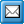﻿ Simple
QPR Knowledge Base 2017.1

SimpleSimpleThis type is used when the model element's attribute is of basic data type. When ModelAttributeType is simple AttributeType XML attribute = "Simple" and there is TypeProperty  DataType = xxx.

Supported data types are:

Boolean, represents Boolean values, which are either true or false.

Int, represents an integer with a minimum value of -2147483648 and maximum of 2147483647.

Double, represents double-precision 64-bit floating-point numbers.

String, represents character strings.

DateTime, represents a specific instance of time. The character format of date and time depends on operating system regional settings. Used format is defined in XML documents root element Model.

Date, represents a calendar date.

Time, represents a specific instance of time that recurs every day.

HexBinary, represents arbitrary hex-encoded binary data.

Example: in QPR Modeling Client the modeler may define the number of units of available resources. When this information is exported to XML its AttributeType is Simple and DataType Double.

<ModelAttributeType Name="Amount Available" AttributeType="Simple">

<TypeProperty Name="DataType" Value="double" />

</ModelAttributeType>

<Attribute AttributeName="Amount Available">

<Value>100</Value>

</Attribute>